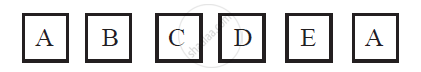# A Child Has a Die Whose Six Faces Shows the Letters as Given Below - Mathematics

A child has a die whose six faces shows the letters as given below:The die is thrown once. What is the probability of getting (i) A? (ii) D?

#### Solution

Total number of possible outcomes on the dice = 6

(i) Total number of faces having A on it = 2

P (getting A) = 2/6 = 1/3

(ii) Total number of faces having D on it = 1

P (getting D) = 1/6

Concept: Probability - A Theoretical Approach
Is there an error in this question or solution?

#### APPEARS IN

NCERT Class 10 Maths
Chapter 15 Probability
Exercise 15.1 | Q 19 | Page 310